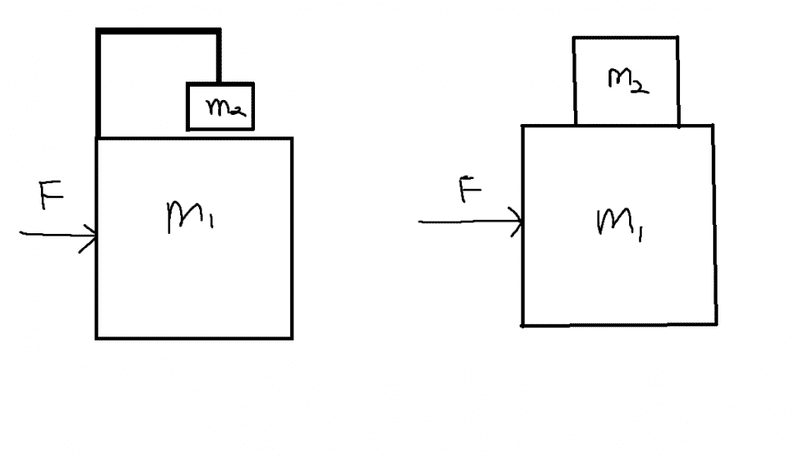# How do I use a free body diagram in this case?In the above cases I want to find the acceleration a for m1. I can do it by considering m1 and m2 to be a system, which would give me a = F/(m1+m2). How can I use a free-body diagram instead to calculate the acceleration? Using a free-body diagram, m2 only affects the vertical motion by exerting a vertical force and not the horizontal acceleration but we know that since the mass of the system has increased the acceleration will decrease. TLDR; how would I solve for the acceleration of m1 in both of the above cases using only a free-body diagram. All surfaces are frictionless.

jtbell
Mentor
Using a free-body diagram, m2 only affects the vertical motion by exerting a vertical force and not the horizontal acceleration

In the first diagram, is the support arm holding m2 rigid, and rigidly attached to m1? It might make things more clear if you consider the support arm as a third object with a very small mass.

In the second diagram, if the contact between m1 and m2 is indeed frictionless, what should you expect to happen, intuitively, when you push on m1 (alone)? Have you ever pushed one object out from underneath another one that is resting on top of it?

In the first diagram, is the support arm holding m2 rigid, and rigidly attached to m1? It might make things more clear if you consider the support arm as a third object with a very small mass.

In the second diagram, if the contact between m1 and m2 is indeed frictionless, what should you expect to happen, intuitively, when you push on m1 (alone)? Have you ever pushed one object out from underneath another one that is resting on top of it?

1) Yes, it's holding m2 in place
2) Yes, it would slide from beneath m2, but for the time m2 is on top of m1, wouldn't it affect the acceleration?

Could you please show me how to make a free-body diagram in the two cases such that the horizontal acceleration is affected too?

For 2) above, no it would not affect the acceleration of m1 because there is no friction so no force.

I'm not sure what you're getting at with your question. My best guess is you want to figure out how much of F is going to accelerate m1 and how much is going to accelerate m2. You can't do that with a free-body diagram in this case.